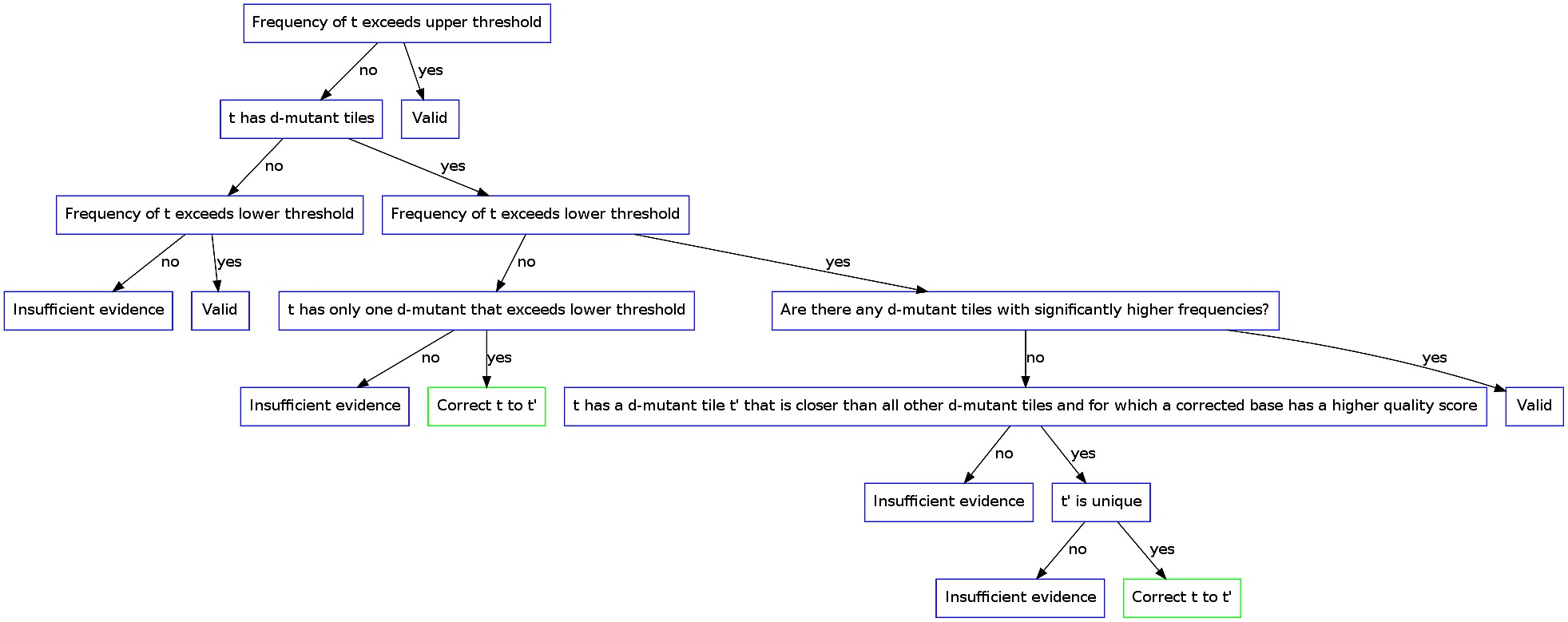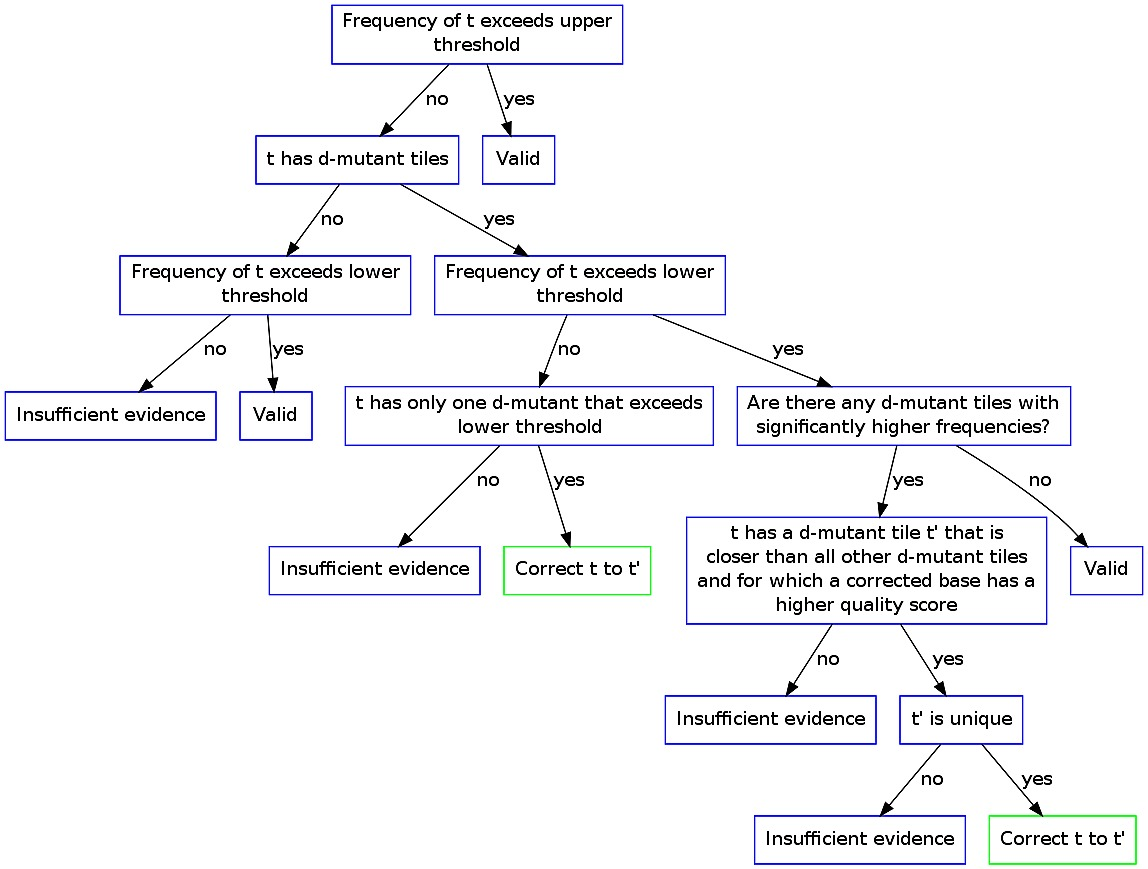# Text wrapping with dot (graphviz)

I used the code below to create a graphic using dot (graphviz).

digraph
{
node [color=Blue,shape=box]

1.1 [label="Frequency of t exceeds upper threshold"]
2.1 [label="t has d-mutant tiles"]
2.2 [label="Valid"]
3.1 [label="Frequency of t exceeds lower threshold"]
3.2 [label="Frequency of t exceeds lower threshold"]
4.1 [label="Insufficient evidence"]
4.2 [label="Valid"]
4.3 [label="t has only one d-mutant that exceeds lower threshold"]
4.4 [label="Are there any d-mutant tiles with significantly higher frequencies?"]
5.1 [label="Insufficient evidence"]
node [color=Green] 5.2 [label="Correct t to t'"] node [color=Blue]
5.3 [label="t has a d-mutant tile t' that is closer than all other d-mutant tiles and for which a corrected base has a higher quality score"]
5.4 [label="Valid"]
6.1 [label="Insufficient evidence"]
6.2 [label="t' is unique"]
7.1 [label="Insufficient evidence"]
node [color=Green] 7.2 [label="Correct t to t'"] node [color=Blue]

1.1 -> 2.1 [label="no"]
1.1 -> 2.2 [label="yes"]
2.1 -> 3.1 [label="no"]
2.1 -> 3.2 [label="yes"]
3.1 -> 4.1 [label="no"]
3.1 -> 4.2 [label="yes"]
3.2 -> 4.3 [label="no"]
3.2 -> 4.4 [label="yes"]
4.3 -> 5.1 [label="no"]
4.3 -> 5.2 [label="yes"]
4.4 -> 5.3 [label="no"]
4.4 -> 5.4 [label="yes"]
5.3 -> 6.1 [label="no"]
5.3 -> 6.2 [label="yes"]
6.2 -> 7.1 [label="no"]
6.2 -> 7.2 [label="yes"]
}


As you can see, some of the boxes in the graphic have a lot of text in the label. I can insert \n characters to make sure the boxes aren't too wide, but I'm wondering if there is a way I can set the width of the boxes and then have the box labels do a hard wrap. Is this possible?

graphviz doesn't support automatic line breaks. You have to put the \n in manually.

you can set a width and a height to a node and define it as fixedsized - this will limit the size of the node and draw only as much text as fits into the node

• Actually for me it turned out to be false, text simply went out of the node shape. Aug 24 '12 at 14:33

Although graphviz does not support text wrapping by itself, dot2tex (latex+graphviz) does. The dot2texi latex package gives an all-in-one solution, with (from the users point of view) a single call to a single tool to build the graph.

A short example:

\documentclass{standalone}
\usepackage{dot2texi}
\usepackage{tikz}
\usetikzlibrary{shapes,arrows}
\begin{document}
\begin{dot2tex}[dot]
digraph G {
d2toptions ="--autosize"
node    [lblstyle="text width=10em,align=center"]
a       [texlbl="This text will be automatically wrapped, for example at a fixed width."]
b       [texlbl="Manual linebreaks from past century can be avoided!"]
a -> b
}
\end{dot2tex}
\end{document}


This can be compiled invoking for example: pdflatex --shell-escape myFile.tex, the text will be automatically wrapped at the prescribed fixed width.

As a side note, this tool seems a handy workaround for graphviz' limited typesetting control of the nodes contents.

• Great answer. Please make sure you have dot2tex installed, or else pdflatex will ask you to do the conversion "by hand". Jan 21 at 14:24

The OP wrote a whole Perl script to achieve this. I found it in his blog: Text wrapping with dot (graphviz).

### ⚠ Note

This only works if the labels are in the format node [ label=”node label” ]. If the nodes are declared directly (e.g. ”node label”) then it doesn’t work

Perl script:

#!/usr/bin/perl
use strict;

my $usage = "setdotlabelwidth [char-width] < [dotfile]"; my$width = shift() or die("Usage: $usage$!");

while(<STDIN>)
{
if(m/label="(.*?)"/)
{
my $labeltext =$1;
my @words = split(/ /, $labeltext); my @newtext = (); my$newline = "";
foreach my $word(@words) { if( length($newline) > 0 and
length($newline) + length($word) > $width ) { push(@newtext,$newline);
$newline = ""; }$newline .= " " if( length($newline) > 0 );$newline .= $word; } push(@newtext,$newline) if( length($newline) > 0 ); my$newlabel = join("\\n", @newtext);
s/label=".*?"/label="\$newlabel"/;
}
print;
}


Save this program as setdotlabelwidth, then simply pipe the output into GraphViz. If for example you want to set the width to 35 characters, then the command is:

./setdotlabelwidth 35 < tile-error-correction.dot | dot -Tpng -o tile-error-correction.png

Before:After: